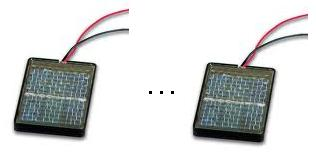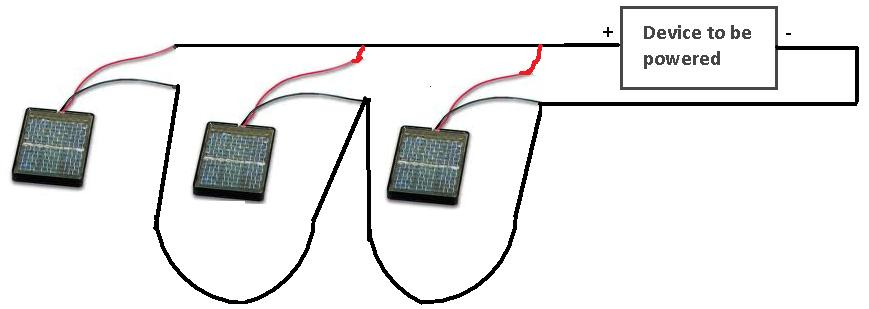﻿ How to Connect Solar Cells in Series and in Parallel# How to Connect Solar Cells in Series and in ParallelIn this article, we will show how to connect solar cells in series and in parallel.

### How to Connect Solar Cells in Series

To connect solar cells in series, you tie the negative terminal of one solar cell to the positive terminal of the next cell and keep on doing this to tie all of the cells in series. This is shown below:When you connect solar cells in series, the voltage of each cell adds up. You increase the net voltage of the circuit. For example, if you tie 3 solar cells together and each has a voltage rating of up to 0.5V, the net voltage will be 1.5V, since the 3 voltages add together. In series, voltages add. Current stays the same. So if each solar cell had a current rating of up to 0.1A in bright light, the circuit. will output 0.1A in series.

### How to Connect Solar Cells in Parallel

To connect solar cells in parallel, you tie all the positive terminals of the cells together to form a common positive connection, and you tie all the negative terminals of the cells together to form a common negative connection. This way, all the cells are in parallel. This is shown below:When you connect solar cells in parallel, the current of each cell adds up. You increase the net current of the circuit. For example, if you tie 3 solar cells together and each has a current rating of up to 0.1A in bright light, the net current will be 0.3A, or 300mA, since the 3 currents add together. In parallel, currents add. Voltage stays the same. So if each solar cell had a voltage rating of 0.5V, the circuit will output 0.5V.